# 1.PTA实验作业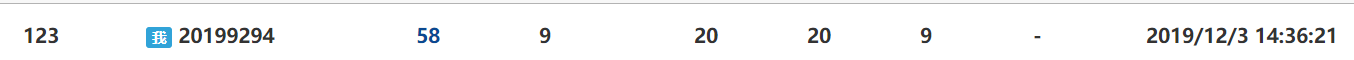## 1.1 题目名1

本题要求实现一个函数，判断任一给定整数N是否满足条件：它是完全平方数，又至少有两位数字相同，如144、676等。

int IsTheNumber ( const int N );

#include <stdio.h>
#include <math.h>

int IsTheNumber ( const int N );

int main()
{
int n1, n2, i, cnt;

scanf("%d %d", &n1, &n2);
cnt = 0;
for ( i=n1; i<=n2; i++ ) {
if ( IsTheNumber(i) )
cnt++;
}
printf("cnt = %d\n", cnt);

return 0;
}

/* 你的代码将被嵌在这里 */

105 500

cnt = 6


### 1.1.1数据处理

数据表达：用了n,i,b,a[],m两个变量,i的值是通过不断循环加1得到,n的值是N的值,m的平方数是N，a[]是一个数组，都是整型变量。

int b,a←{0},m←sqrt(N),i,n←N; /*定义五个变量i，n，b,a,m*/
IF m*m=n THEN                    /*判断n是否是完全平方数*/
do while n                       /*在循环里判断平方数是否至少有两位数字相同*/
do b←n%10
a[b]←a[b]+1
n←n/10
for i←0 to 9
do IF a[i]>1 THEN             /*通过数组判断相同数的个数*/
do return 1
return 0


### 1.1.2实验代码截图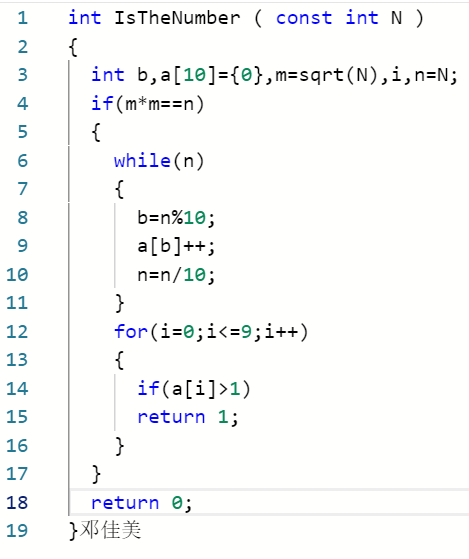### 1.1.3 造测试数据

105 500 cnt = 6 与题目输出样例一样，正确
40 600 cnt = 7 随机取数，检验代码是否正确
209 579 cnt = 4 随机取数，检验代码是否正确

### 1.1.4 PTA提交列表及说明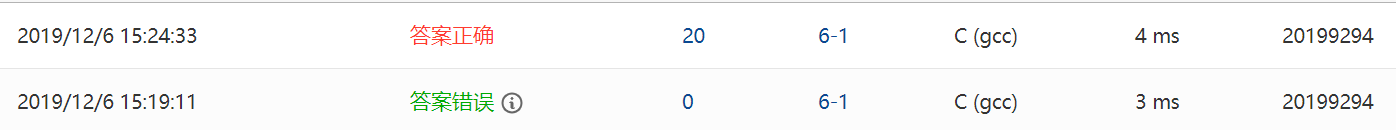1.答案错误：把b=n%10中的n打成了N，改正过来后正确了
2.答案正确。


## 1.2 题目2

本题要求实现两个函数：一个函数判断给定正整数的各位数字之和是否等于5；另一个函数统计给定区间内有多少个满足上述要求的整数，并计算这些整数的和。

int is( int number );
void count_sum( int a, int b );

count = 满足条件的整数个数, sum = 这些整数的和

#include <stdio.h>

int is( int number );
void count_sum( int a, int b );

int main()
{
int a, b;

scanf("%d %d", &a, &b);
if (is(a)) printf("%d is counted.\n", a);
if (is(b)) printf("%d is counted.\n", b);
count_sum(a, b);

return 0;
}

/* 你的代码将被嵌在这里 */

104 999

104 is counted.
count = 15, sum = 3720


### 1.2.1 数据处理

数据表达：用了number,count,sum,a,b五个变量；a,b的值是通过输入得到；number的值代表a，b的值；count的值是通过for循环得到；sum是通过循环和式子sum+=a得到的；都是整型变量

int number;
IF number%10+(number/10)%10+(number/100)%10+(number/1000)%10=5 THEN /*判断给定正整数的各位数字之和是否等于5*/
do return 1
else
return 0
int a,b,count←0,sum←0;
for a←a to b                                                        /*统计给定区间内有多少个满足上述要求的整数，并计算这些整数的和*/
do IF is(a)=1 THEN
do count←count+1
sum←sum+a
printf("count ← %d, sum ← %d",count,sum);


### 1.2.2 实验代码截图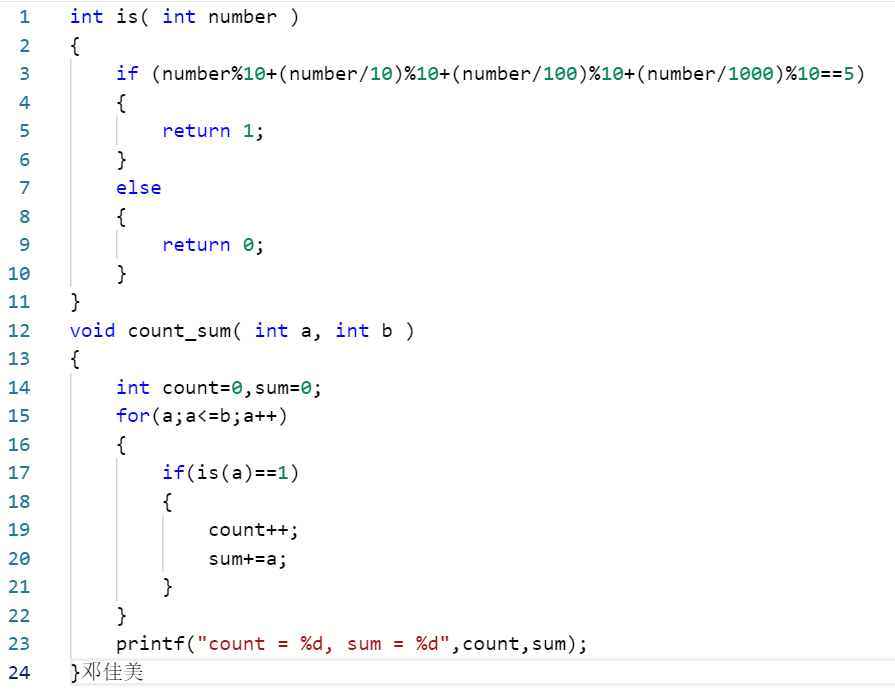### 1.2.3 造测试数据

104 999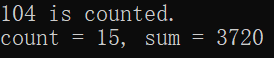与题目输出样例一样，正确
672 8092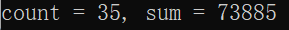取1-10000之间的数，输入的数各位数之和不等于5
500 398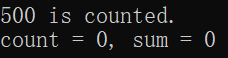a>b,不符合题意

### 1.2.4 PTA提交列表及说明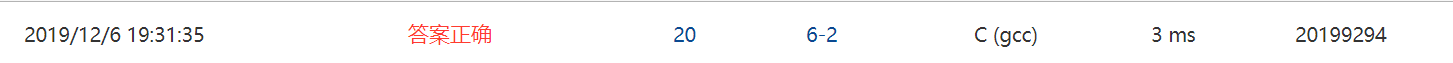1.答案正确。通过number%10+(number/10)%10+(number/100)%10+(number/1000)%10把正整数分成个位数相加判断是否之和等于5，用for循环得出count，sum。


# 2.代码互评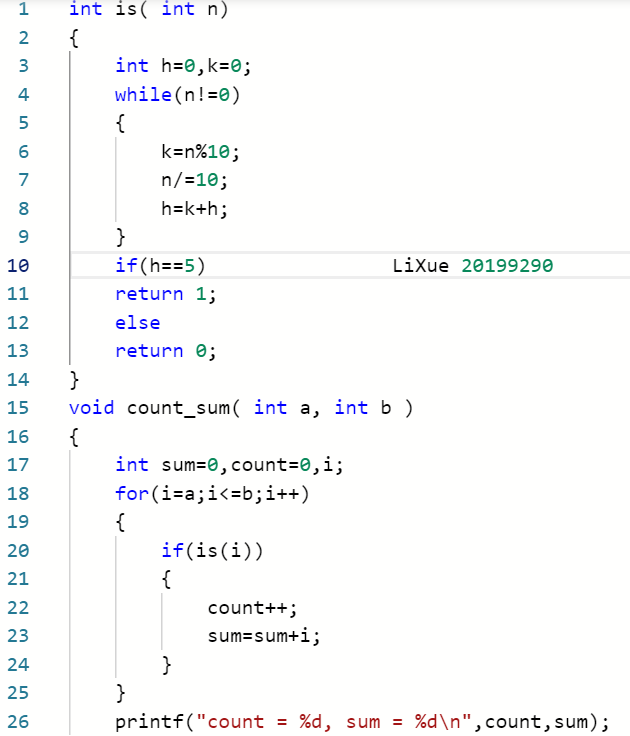同学1在判断给定正整数的各位数字之和是否等于5时用了while循环和if语句判断，while循环中通过k=n%10,n/=10,h=k+h把n中的数分成个位数，然后相加在if语句里面判断。我只用了一个if语句判断，条件是number%10+(number/10)%10+(number/100)%10+(number/1000)%10==5，满足了就可以进行下一步了。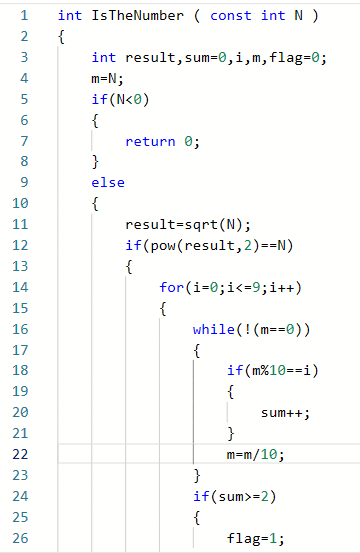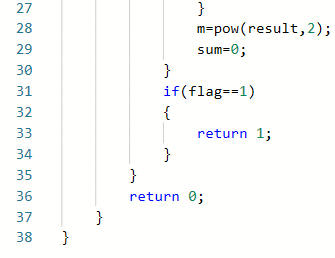同学2的代码没有用数组，先用if-else语句判断N的正负，然后在else语句里面嵌套了if语句，用来判断N是否是完全平方数，又用了for循环，while循环判断N是否至少有两位数字相同。同学2的代码比较长，不过没有用数组，易懂些。


# 3.学习总结

## 3.2 累积代码行和博客字数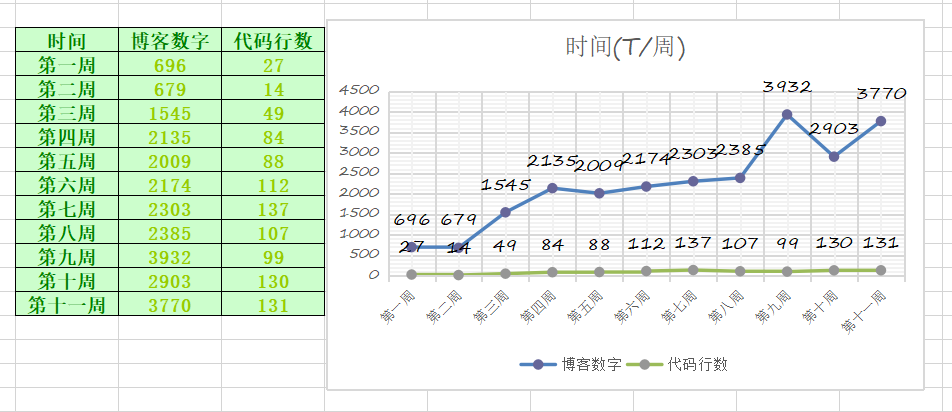## 3.3 学习内容总结和感悟

### 3.3.1 学习内容总结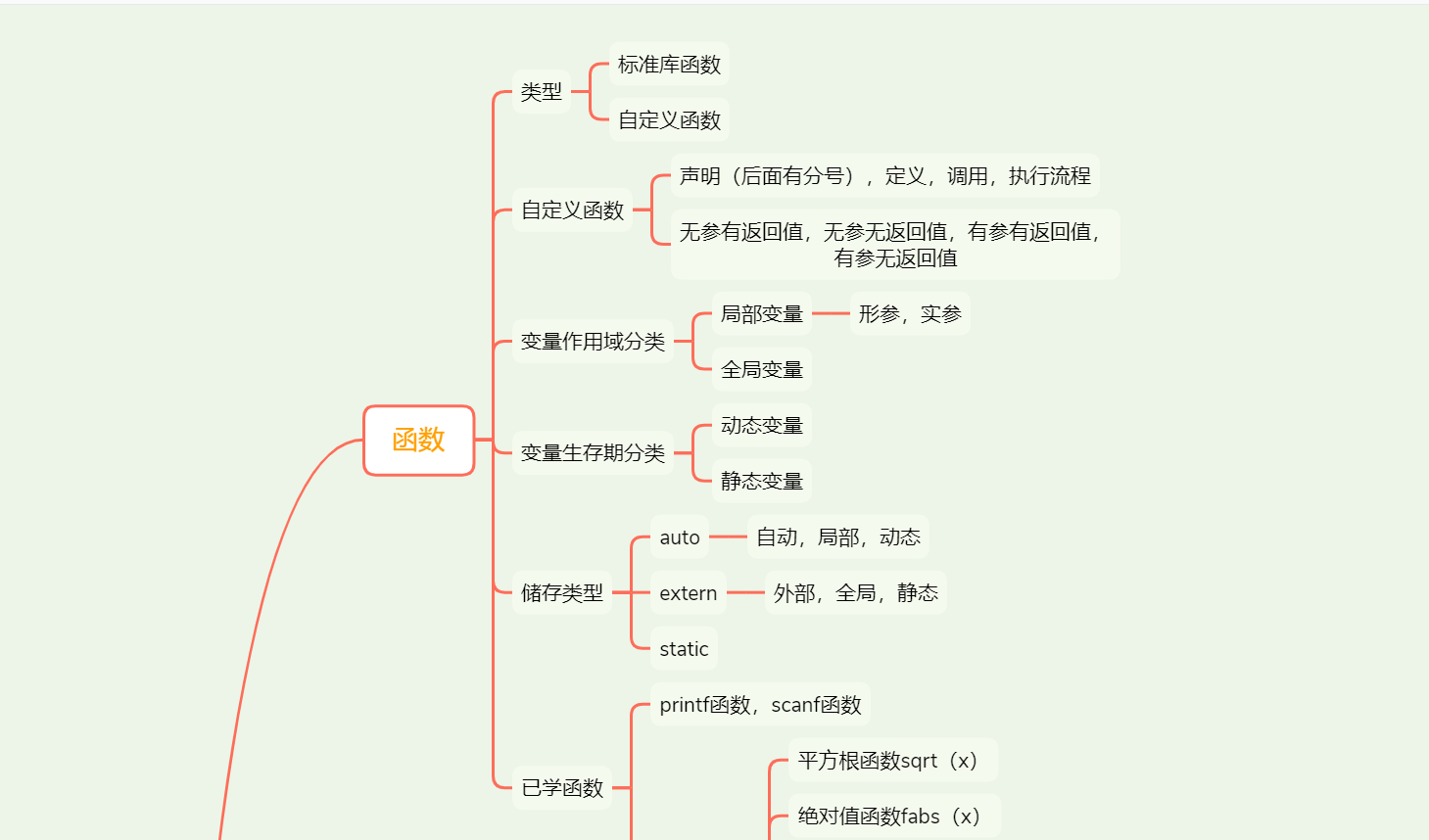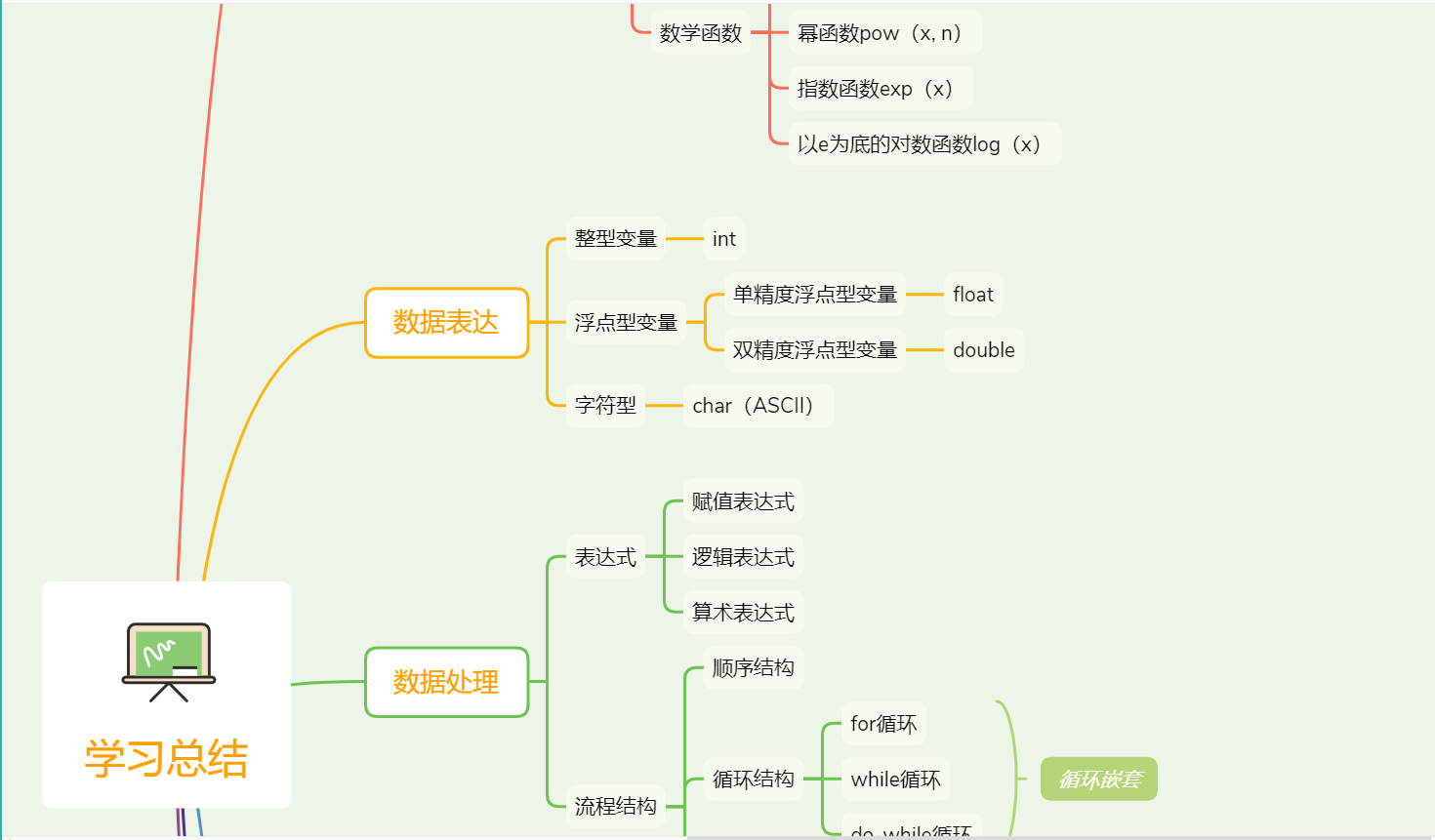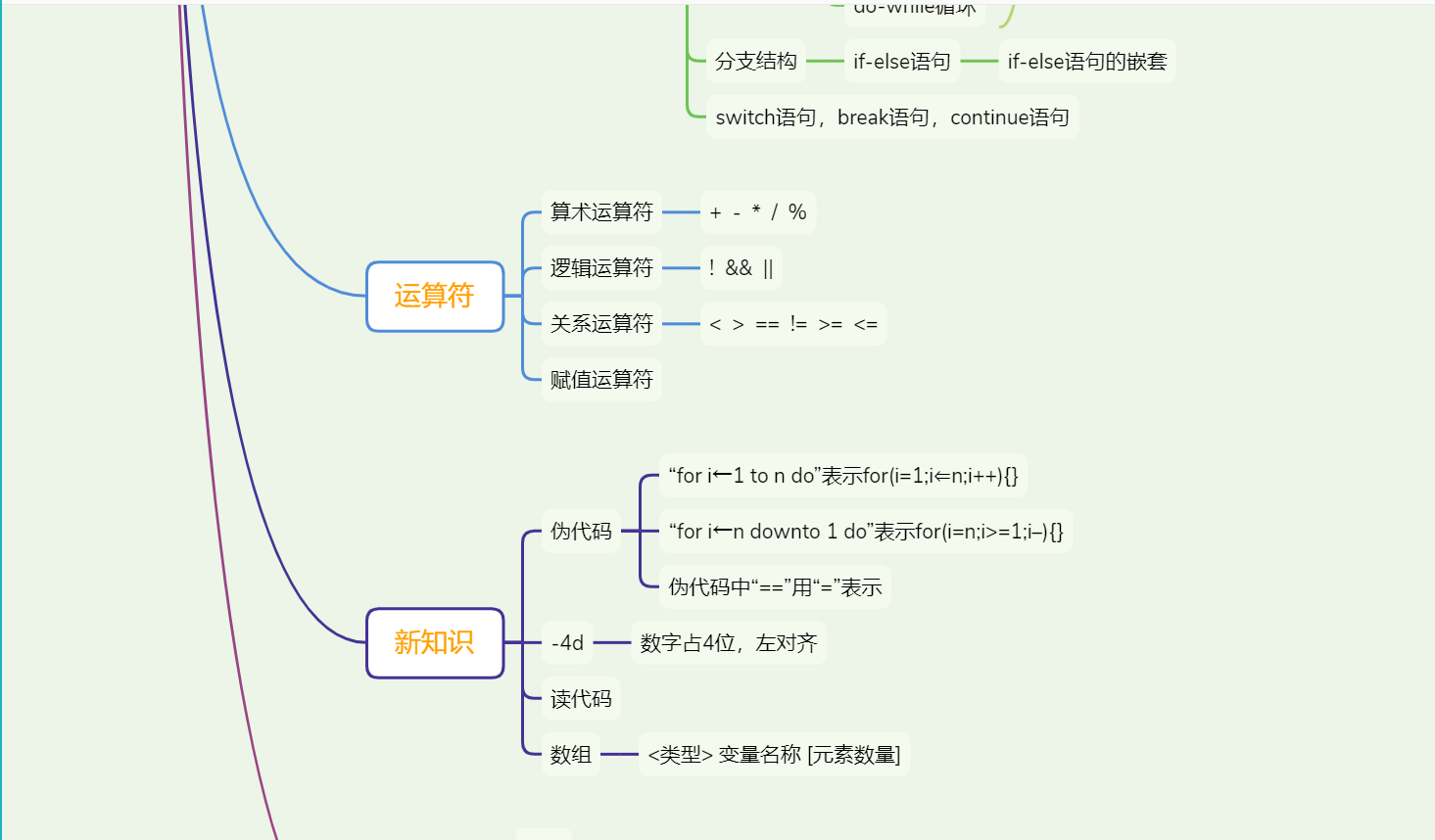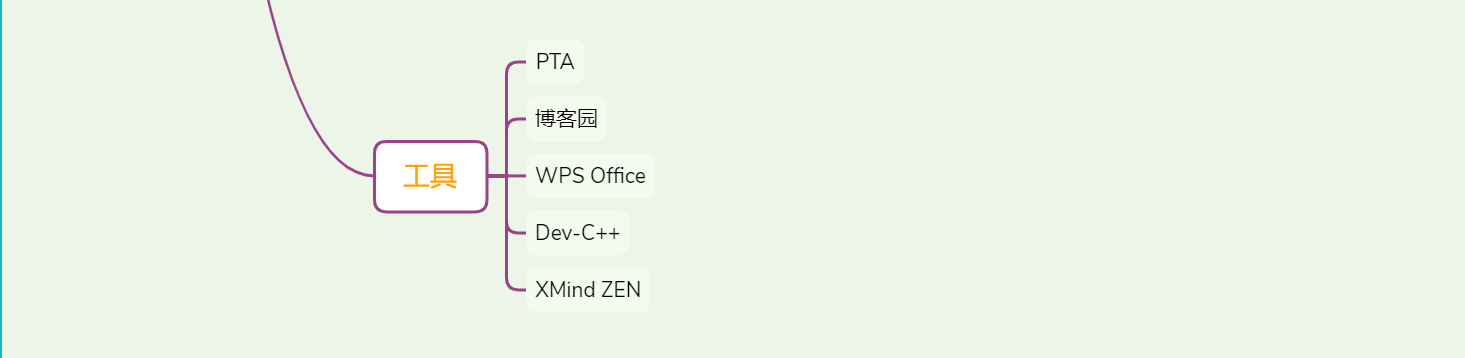### 3.3.2 学习体会

这次写函数题的时候我都在想我之前是怎么做的，感觉有点模糊，但后面做了后又觉得我会做。不能那么早就被吓到了。读清题意，捋清思绪。虽然我有的是问同学的，哈哈。不过编程题我没怎么挑战，等有时候在做了。在写PTA前看了有关数组的知识，以为差不多懂了，可是用的时候，就懵逼了。还是要多实践的好！
posted @ 2019-12-06 17:51  邓佳美  阅读(145)  评论(1编辑  收藏